## 通用的优化方法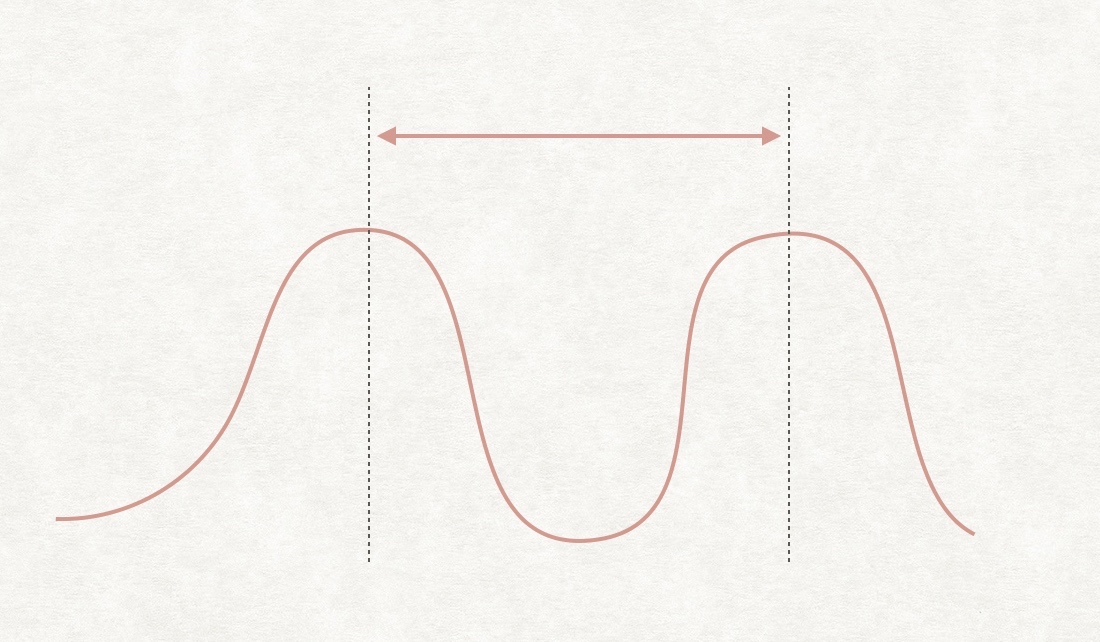left, right := trimLeftRight(heights)
if left >= right {
return res
}

func trimLeftRight(heights []int) (int, int) {
n := len(heights)
left, right := 0, n-1
for left < right && heights[left] <= heights[left+1] {
heights[left] = 0
left++
}
for right > left && heights[right] <= heights[right-1] {
heights[right] = 0
right--
}
return left, right
}


## 解法一：暴力（遍历不同的高度）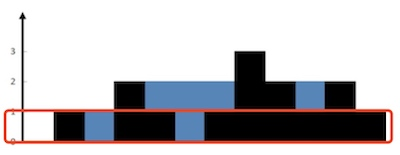315/315 cases passed (960 ms)
Your runtime beats 5.14 % of golang submissions
Your memory usage beats 100 % of golang submissions (2.8 MB)


func trap(heights []int) int {
if len(heights) == 0 {
return 0
}
n := len(heights)
m := 0
for _, h := range heights {
m = Max(m, h)
}
res := 0
left, right := 0, n-1
for ; m > 0; m-- {
left, right = trimLeftRight(heights)
if left >= right {
return res
}
for i := left; i <= right; i++ {
if heights[i] == 0 {
res++
} else {
heights[i]--
}
}
}
return res
}


## 解法二：暴力（遍历不同的柱子）

Min(height[left], height[right]) - height[i]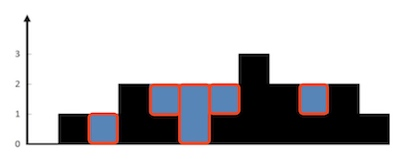315/315 cases passed (80 ms)
Your runtime beats 11.68 % of golang submissions
Your memory usage beats 75.85 % of golang submissions (2.8 MB)


func trap(heights []int) int {
if len(heights) == 0 {
return 0
}

left, right := trimLeftRight(heights)
if left >= right {
return 0
}

res := 0
for i := left + 1; i <= right-1; i++ { // 左右边界上一定没雨水
hLeft, hRight := 0, 0
for j := left; j < i; j++ {
hLeft = Max(hLeft, heights[j])
}
for j := i + 1; j <= right; j++ {
hRight = Max(hRight, heights[j])
}
h := heights[i]
if hLeft > h && hRight > h {
res += Min(hLeft, hRight) - h
}
}
return res
}


## 解法三：使用线段树优化解法二

315/315 cases passed (8 ms)
Your runtime beats 22.64 % of golang submissions
Your memory usage beats 5.1 % of golang submissions (4.3 MB)


func trap(heights []int) int {
if len(heights) == 0 {
return 0
}
left, right := trimLeftRight(heights)
if left >= right {
return 0
}

res := 0
sTree := BuildSegmentTree(heights, 0, len(heights)-1) // 1. 构建线段树
for i := left + 1; i <= right-1; i++ {                // 左右边界上一定没雨水
hLeft := sTree.Query(left, i-1) // 2. 使用 Query() 方法查找区间最值
hRight := sTree.Query(i+1, right)
h := heights[i]
if hLeft > h && hRight > h {
res += Min(hLeft, hRight) - h
}
}
return res
}

type SegmentTreeNode struct {
start int
end   int
max   int
left  *SegmentTreeNode
right *SegmentTreeNode
}

func BuildSegmentTree(nums []int, left, right int) *SegmentTreeNode {
if left > right {
return nil
}
root := &SegmentTreeNode{left, right, nums[left], nil, nil} // 根据节点区间的左边界值初始化
if left == right {
return root
}
mid := (left + right) / 2
root.left = BuildSegmentTree(nums, left, mid)
root.right = BuildSegmentTree(nums, mid+1, right)
root.max = Max(root.left.max, root.right.max)
return root
}

func (root *SegmentTreeNode) Query(start, end int) int {
if start <= root.start && end >= root.end {
return root.max
}
if start > root.end || end < root.start {
return math.MinInt32
}
return Max(root.left.Query(start, end), root.right.Query(start, end))
}

func Min(a, b int) int {
if a > b {
return b
}
return a
}

func Max(a, b int) int {
if a > b {
return a
}
return b
}


## 解法四：使用动态规划优化解法二

maxLeft[i] = Max(maxLeft[i-1], heights[i-1])
maxRight[i] = Max(maxRight[i+1], heights[i+1])


315/315 cases passed (0 ms)
Your runtime beats 100 % of golang submissions
Your memory usage beats 14.63 % of golang submissions (3.1 MB)


func trap(heights []int) int {
if len(heights) == 0 {
return 0
}
left, right := trimLeftRight(heights)
if left >= right {
return 0
}

n := len(heights)
res := 0

maxLeft, maxRight := make([]int, n), make([]int, n)
maxLeft, maxRight[n-1] = 0, 0
for i := 1; i < n; i++ {
maxLeft[i] = Max(maxLeft[i-1], heights[i-1])
}
for i := n - 2; i >= 0; i-- {
maxRight[i] = Max(maxRight[i+1], heights[i+1])
}

for i := left + 1; i <= right-1; i++ { // 左右边界上一定没雨水
hLeft, hRight := maxLeft[i], maxRight[i]
h := heights[i]
if hLeft > h && hRight > h {
res += Min(hLeft, hRight) - h
}
}
return res
}


## 解法五：使用双指针优化解法四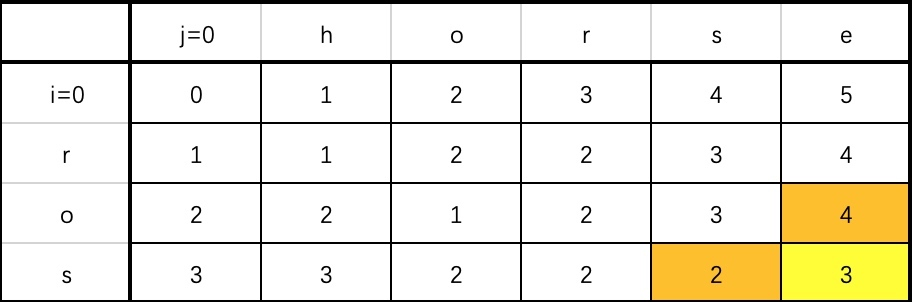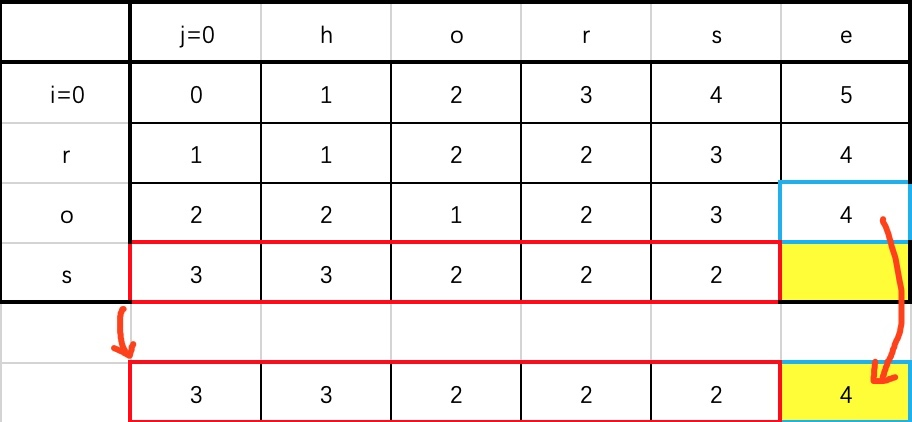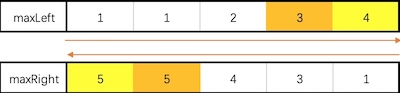maxRight := make([]int, n) // maxRight 还是一个一维数组
maxRight[n-1] = heights[n-1]
for i := n-2; i >= 0; i-- { // 需要提前从右往左遍历，求出每个 maxRight[i]
maxRight[i] = Max(maxRight[i+1], heights[i+1])
}
maxLeft := heights // maxLeft 压缩为一个变量
for i := 1; i < n; i++ {
maxLeft = Max(maxLeft, heights[i-1])
// ...
}


func trap(heights []int) int {
if len(heights) == 0 {
return 0
}
left, right := trimLeftRight(heights)
if left >= right {
return 0
}

res := 0
left, right = left+1, right-1                          // 左右边界一定无法接雨水
maxLeft, maxRight := heights[left-1], heights[right+1] // 初始化
for left <= right {
if maxLeft <= maxRight { // 从左向右
h := heights[left]
if maxLeft > h {
res += maxLeft - h
}
maxLeft = Max(maxLeft, h)
left++
} else { // 从右向左
h := heights[right]
if maxRight > h {
res += maxRight - h
}
maxRight = Max(maxRight, h)
right--
}
}
return res
}


## 解法六：单调栈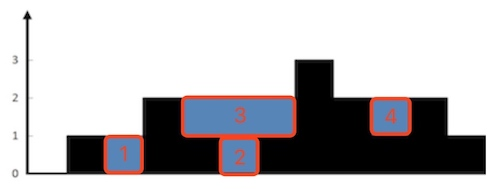left = stack[top-1]right = itop = stack[top]，如下图所示，可以计算出 leftrighttop 三根柱子包围的雨水量为：

(Min(heights[left], heights[right]) - heights[top]) × (right-left-1)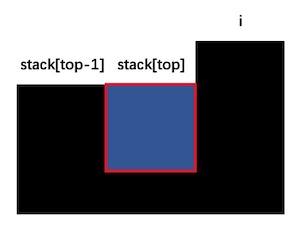1. 从左到右遍历所有元素
2. 如果当前元素的高度小于等于栈顶元素的高度，就将当前下标入栈
3. 否则，不断将栈顶元素出栈，同时根据上述公式计算雨水量
4. 重复第 3 步，直到当前元素的高度不大于栈顶元素的高度，然后将当前下标入栈

func trap(heights []int) int {
if len(heights) == 0 {
return 0
}
left, right := trimLeftRight(heights)
if left >= right {
return 0
}

res := 0
stack := NewStack()
for i := left; i <= right; i++ { // 从 1 开始遍历
for !stack.Empty() && heights[i] > heights[stack.Top()] {
h := heights[stack.Pop()] // 弹出栈顶元素
if stack.Empty() {        // 弹出元素后栈不能为空
break
}
left, right := stack.Top(), i
res += (Min(heights[left], heights[right]) - h) * (right - left - 1)
}
stack.Push(i)
}
return res
}

// 以下是栈的模板代码

type ElementType = int
type Stack struct {
s []ElementType
}

func NewStack() *Stack {
return &Stack{
s: make([]ElementType, 0),
}
}

func (s *Stack) Empty() bool {
return len(s.s) == 0
}

func (s *Stack) Top() ElementType {
if s.Empty() {
return 0
}
return s.s[len(s.s)-1]
}

func (s *Stack) Pop() ElementType {
if s.Empty() {
return 0
}
t := s.s[len(s.s)-1]
s.s = s.s[:len(s.s)-1]
return t
}

func (s *Stack) Push(v ElementType) {
s.s = append(s.s, v)
}